# Functions Exercise 1c Solutions ||TS||## Functions Exercise 1c Solutions

The famous mathematician ” Lejeune Dirichlet”  defined a function.

Function: A variable is a symbol which represents any one of a set of numbers, if two variables x and y so related that whenever a value is assigned to x there is autometically assigned by some rule or correspondence a value to y, then we say y is a function of x.

Chapter 1 Functions Exercise 1c Solutions for inter first year  students, prepared by Mathematics expert of www.basicsinmaths.com

## Exercise 1c

Exercise 1(c) Solutions

### I.

#### 1.Find the domains of the following real valued functions

(i)

f (x) =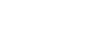given function is f (x) =f (x) is defined when (x2 – 1) (x + 3) ≠ 0

⟹ (x2 – 1) ≠ 0 or (x + 3) ≠ 0

⟹ (x + 1) (x – 1) ≠ 0 or (x + 3) ≠ 0

⟹ x ≠ 1, x ≠ – 1 or x ≠ – 3

∴ Domain of f(x) is R – {– 1, – 3, 1}

(ii)

f (x) =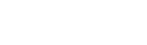Given function is f (x) =f (x) is defined when (x – 1) (x – 2) (x – 3) ≠ 0

⟹ x ≠ 1, x ≠ 2 or x ≠ 3

∴ Domain of f(x) is R – {1, 2, 3}

(iii)

f (x) =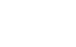Given function is f (x) =f (x) is defined when 2 – x > 0 and 2 – x ≠ 1

⟹ 2 > x  and  2 – 1 ≠ x

⟹ 2 > x  and  x ≠ 1

∴ Domain of f(x) is (– ∞, 2) – {1}

(iv)

f (x) =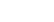Given function is f (x) =f (x) is defined when x ∈ R

∴ Domain of f(x) is R

Functions Exercise 1c

(v)

f (x) =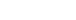Given function is f (x) =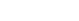f (x) is defined when 4x – x2 ≥ 0

⟹ x (4 – x) ≥ 0

⟹ x (x – 4) ≤ 0

⟹ (x – 0) (x – 4) ≤ 0

⟹ x ∈ [0, 4]

∴ Domain of f(x) is [0, 4]

(vi)

f (x) =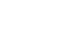Given function is f (x) =f (x) is defined when 1 – x2 > 0

⟹ x2 – 1 < 0

⟹ (x – 1) (x + 1) < 0

⟹ x ∈ (– 1, 1)

∴ Domain of f(x) is (– 1, 1)

(vii)

f (x) =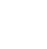Given function is f (x) =f (x) is defined when x + 1≠ 0

⟹ x ≠ – 1

∴ Domain of f(x) is R – {– 1}

(viii)

f(x) =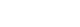Given function is f (x) =f (x) is defined when x2 – 25 ≥ 0

⟹ (x – 5) (x + 5) ≥ 0

⟹ x ∈ (–∞, –5] ∪ [5, ∞)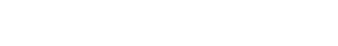⟹ x ∈ R – (– 5, 5)

∴ Domain of f(x) is R – (– 5, 5)

Functions Exercise 1c

(ix)

f(x) =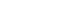Given function is f (x) =f (x) is defined when x – [x] ≥ 0

⟹ x ≥ [x]

⟹ x ∈ R

∴ Domain of f(x) is R

(x)

f(x) =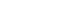Given function is f (x) =f (x) is defined when [x] – x ≥ 0

⟹ [x] ≥ x

⟹ x ∈ Z

∴ Domain of f(x) is Z

Functions Exercise 1c

##### 2. find the ranges of the following real valued functions

(i)

f(x) =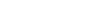Given function is f (x) =Let y =⟹  |4 – x2| = ey

∵ ey > 0 ∀ y ∈ R

∴ Range of f(x) is R

(ii)

f(x) =Given function is f (x) =f (x) is defined when [x] – x ≥ 0

⟹ [x] ≥ x

⟹ x ∈ Z

Domain of f(x) is Z

Range of f = {0}

(iii)

f(x) =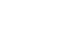Given function is f (x) =f (x) is defined when x ∈ R

Domain of f(x) is R

For x ∈ R   [x] is an integer

Since sin nπ = 0, ∀ n ∈ z

⟹ sin π[x] = 0

∴ Range of f = {0}

(iv)

f (x) =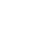Given function is f (x) =f (x) is defined when x – 2 ≠ 0

⟹ x ≠ 2

Domain of f(x) is R – {2}

Let y ==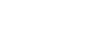= x + 2

If x = 2 ⟹ y = 2 + 2 = 4

∴ Range of f(x) is R – {4}

Functions Exercise 1c

(v)

f (x) =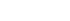let y =y2 = 9 + x2

x2 = y2 – 9

x =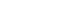it is defined when y2 – 9 ≥ 0

⟹ (y – 3) (y + 3) ≥ 0

y ∈ (– ∞, – 3] ∪ [3, ∞)

but y =≥ 0

∴ Range of f(x) is [3, ∞)

##### find

Sol:

Given f and g are real valued functions f(x) = 2x – 1 ang g (x) = x2

(i)

(3f – 2g) (x) = 3 f(x) – 2g (x)

= 3 (2x – 1) – 2(x2)

= 6x – 3 – 2x2

= – 2x2 + 6x – 3

∴ (3f – 2g) (x) =– 2x2 + 6x – 3

(ii)

(fg) (x) = f (x) g (x)

= (2x – 1) (x2)

= 2x3 + x2

∴ (fg) (x) = 2x3 + x2

(iii)##### 4. If f = {(1, 2), (2, – 3) (3, – 1)} then find (i) 2f (ii) (fog) 2 + f iii) f2 (iv)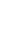Sol:

Given f = {(1, 2), (2, – 3) (3, – 1)}

(i)

(2f) (1) = 2 f (1) = 2 × 2 = 4

(2f) (2) = 2 f (2) = 2 × – 3 = – 6

(2f) (3) = 2 f (3) = 2 × – 1 = – 2

∴ 2f = {(1, 4), (2, – 6) (3, – 2)}

(ii)

(2 + f) (1) = 2 + f (1) = 2 + 2 = 4

(2 + f) (2) = 2 + f (2) = 2 + (– 3) = – 1

(2 + f) (3) = 2 + f (3) = 2 + (– 1) = 1

∴ 2 + f = {(1, 4), (2, – 1) (3, 1)}

(iii)

(f2) (1) = [f (1))]2 = 22 = 4

(f2) (2) = [f (2))]2 = (– 3)2 = 9

(f2) (3) = [f (1))]2 = (– 1)2 = 1

∴ f2= {(1, 4), (2, 9) (3, 1)}

(iv)### II.

#### 1.Find the domain of the following real valued functions

(i)

f (x) =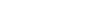f(x) is defined when x2 – 3x + 2 ≥ 0

x2 – 2x – x + 2 ≥ 0

x (x – 2) – 1(x – 2) ≥ 0

(x – 1) (x – 2) ≥ 0

x ∈ (– ∞, 1] ∪ [2, ∞)

∴ Domain of f(x) is R – (1, 2)

(ii)

f(x) = log (x2 – 4x + 3)

f(x) is defined when x2 – 4x + 3 > 0

x2 – 3x – x + 3 > 0

x (x – 3) – 1(x – 3) > 0

(x – 1) (x – 3) > 0

x ∈ (– ∞, 1) ∪ (3, ∞)

∴ Domain of f(x) is R – [1, 3]

(iii)

f(x) =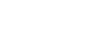f(x) is defined when 2 + x ≥ 0, 2 – x ≥ 0  and x ≠ 0

x ≥ – 2, x ≤ 2 and x ≠ 0

– 2 ≤ x ≤ 2 and x ≠ 0

x ∈ [–2, 2] – {0}

∴ Domain of f(x) is [–2, 2] – {0}

(iv)

f(x) =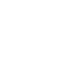f(x) is defined in two cases as follows:

case (i) 4 – x2 ≥ 0 and [x] + 2 > 0

x2 – 4 ≤ 0 and [x] > –2

(x – 2) (x + 2) ≤ 0 and [x] > –2

x ∈ [– 2, 2] and x ∈ [– 1, ∞)

x ∈ [– 1, 2]

case (ii) 4 – x2 ≤ 0 and [x] + 2 < 0

x2 – 4 ≥ 0 and [x] < –2

(x – 2) (x + 2) ≥ 0 and [x] < –2

x ∈ (– ∞, –2] ∪ [2, ∞) and x ∈ (–∞, –2)

x ∈ (–∞, –2)

from case (i) and case (ii)

x ∈ (–∞, –2) ∪ [– 1, 2]

∴ Domain of f(x) is (–∞, –2) ∪ [– 1, 2]

(v)

f(x) =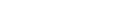f(x) is defined when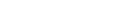≥ 0 and x – x2 > o

x – x2 ≥ (0.3)0   and x2 – x < 0

x – x2 ≥ 1 and x (x – 1) < 0

x2 –x + 1 ≤ 0 and (x – 0) (x – 1) < 0

it is true for all x ∈ R and x ∈ (0, 1)

∴ domain of f(x) =  R∩ (0, 1) = (0, 1)

(vi)

f(x) =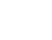f(x) is defined when x +|x| ≠ 0

|x| ≠ – x

|x| ≠ – x

⟹|x| = x

⟹ x > 0

x ∈ (0, ∞)

∴ Domain of f(x) is (0, ∞)

#### 2. Prove that the real valued function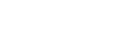is an even function

Sol:

Given f(x) =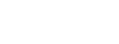#### 3. Find the domain and range of the following functions

(i)

f (x) =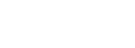Given f(x) =Since [x] is an integer

sin π[x] = tan π[x] = 0 ∀ x ∈ R

∴ domain of f(x) is R

and

since tan π[x] = 0

Range of f(x) = {0}

(ii)

f(x) =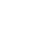Given f (x) =It is defined when 2 – 3x ≠ 0

⟹ 2 ≠ 3x

⟹ x ≠ 2/3

∴ Domain of f (x) = R – {2/3}

Let y = f(x)

y =⟹ y (2 – 3x) = x

2y – 3xy = x

2y = x + 3xy

2y = x (1 + 3y)

⟹ x =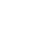It is defined when 1 + 3y ≠ 0

1 ≠ –3y

y ≠ – 1/3

∴ Range of f (x) = R – {– 1/3}

(iii)

f(x) = |x| + | 1 + x|

Given function is f (x) = |x| + |1 + x|

f (x) is defined for all x ∈ R

∴ domain of f(x) = Rf (– 3) = |– 3| + |1 – 3|

=|– 3| + |– 2|

= 3 + 2 = 5

f (– 2) = |– 2| + |1 – 2|

=|– 2| + |– 1|

= 2 + 1 = 3

f (– 1) = |– 1| + |1 – 1|

=|– 1| + |0|

= 1 + 0 = 1

f (0) = |0| + |1 + 0| = 1

f (1) = |1| + |1 + 1|

= 1 + |2|

= 1 + 2 = 3

f (2) = |2| + |1 + 2|

= |2| + |3|

= 2 + 3 = 5

f (3) = |3| + |1 + 3|

= |3| + |4|

= 3 + 4 = 7

∴ Range of f(x) = [1, ∞)

##### Visit my Youtube Channel: Click on Below Logo